# Information, exactness of reproducibility of

(diff) ← Older revision | Latest revision (diff) | Newer revision → (diff)

A measure of the quality of information transmission from an information source (cf. Information, source of) to a receiver (addressee) over a communication channel. The criteria relevant to the exactness of reproducibility of information in the theory of information transmission are usually treated statistically, by isolating the classof admissible joint distributions for pairs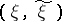in the set of all probability measures on the product, where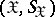is the measurable space of values of a communicationgenerated by the source, and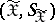is the measurable space of values of the communication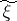received. Exactness of reproducibility of information is often defined in terms of a distortion measure,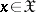,, which is a non-negative measurable function ofand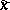. The set of admissible communicationsis then specified by the formula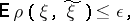(1)

for a given.

In particular, when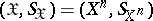and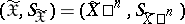, one often uses a componentwise condition for the exactness of reproducibility of information, namely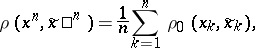where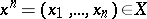,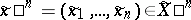,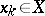,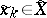,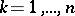, and where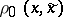,,, is again a non-negative measurable function. In this case, instead of condition (1) one sometimes uses the following condition:(2)

In the case when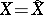and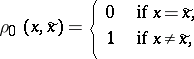the conditions (1) and (2) turn into restrictions on the mean or maximal probability of erroneous decoding (cf. Erroneous decoding, probability of) of separate components of the communication, respectively. In the case of sources with continuous spaces (such as a Gaussian source), it is often assumed that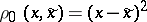.

How to Cite This Entry:
Information, exactness of reproducibility of. Encyclopedia of Mathematics. URL: http://encyclopediaofmath.org/index.php?title=Information,_exactness_of_reproducibility_of&oldid=11964
This article was adapted from an original article by R.L. DobrushinV.V. Prelov (originator), which appeared in Encyclopedia of Mathematics - ISBN 1402006098. See original article